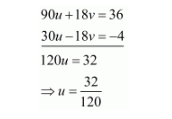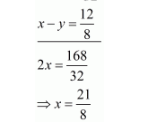# Solve the following systems of equations:

Question:

Solve the following systems of equations:

$\frac{10}{x+y}+\frac{2}{x-y}=4$

$\frac{15}{x+y}-\frac{9}{x-y}=-2$

Solution:

The given equations are:

$\frac{10}{x+y}+\frac{2}{x-y}=4$

$\frac{15}{x+y}-\frac{9}{x-y}=-2$

Let $\frac{1}{x+y}=u$ and $\frac{1}{x-y}=v$ then equations are

$10 u+2 v=4 \ldots(i)$

$15 u-9 v=-2 \ldots(i i)$

Multiply equation $(i)$ by 9 and equation $(i i)$ by 2 and add both equations, we getPut the value of $u$ in equation $(i)$, we get

$10 \times \frac{32}{120}+2 v=4$

$\Rightarrow 2 v=\frac{16}{12}$

$\Rightarrow v=\frac{8}{12}$

Then

$\frac{1}{x+y}=\frac{32}{120}$

$\Rightarrow x+y=\frac{120}{32} \quad \ldots(i i i)$

$\frac{1}{x-y}=\frac{8}{12}$

$\Rightarrow x-y=\frac{12}{8} \ldots(i v)$

$x+y=\frac{120}{32}$Put the value of $x$ in equation $(i i i)$ we get

$1 \times \frac{21}{8}+y=\frac{120}{32}$

$\Rightarrow y=\frac{9}{8}$

Hence the value of $x=\frac{21}{8}$ and $y=\frac{9}{8}$.